# 4.0 Galactic Proper Motion

Before proceeding further, another known value must be transferred to it's galactic equivalent. As I mentioned at the beginning of Chapter 3.0, every star has some movement relative to our Sun. This means that, unless it appears to be traveling directly toward us or away from us, the movement the star makes will be a lateral movement across the sky. Now granted this movement is so small, that no star makes any change that we can see with our naked eye in the course of a single lifetime. Nevertheless, the stars do move and many do relative motion to us to the speed of hundreds of kilometers per second. This motion is a known stellar value and is called Proper Motion.In order to align our coordinate system to galactic polar we must take the given stellar Proper Motion based on the Earth pole and change it into the Proper Motion based on the galactic pole. This can be shown with a trigometric solution.

`                                                            4.0.1`
Galactic Proper Motion
Source Data In Data Out
known  1) The Earth polar declination of the star (d)
known  2) The Earth polar declination of the galactic north (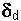)
known  3) The Earth polar angle of Right Ascension of the galactic north (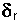)
known  4) Stellar angle of Proper Motion based on Earth polar (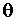) Stellar angle of Proper Motion based on galactic polar (G)

`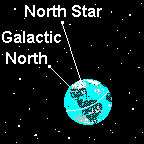Illustration 4.0.2`
Gmay be found from the above values. The Gdiffers from the, because the Earth pole and galactic pole point to different zeniths and therefore any angle relating to one pole will almost always be a different angle relative to the other pole. Since we are dealing with a simple directional angle between 0° and 360°, we shall only need only to add or subtract an angle to change from Earth polar to galactic polar. However, the amount of the angle adjustment will vary, depending on where the star is in the sky.

`                                                           Graph 4.0.3`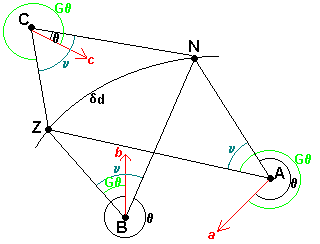Where stars A, B, and C show sample stellar positions looking up from the Earth at both the Earth polar zenith, N, and the galactic polar zenith, Z. The red arrows indicate the direction of the star's movement or "direction of Proper Motion" shown by the small red letters, a, b and c. This angle of this direction is defined by drawing a line from the star to the North Pole, N and taking the angle clockwise around to the red line, the direction of the star's movement. This is shown on the graph as the small black arcs around each star and is a known value of the star commonly called the "angle of Proper Motion". For this paper it will be further clarified as the "Stellar angle of Proper Motion based on Earth polar" and designated as.

My purpose here is to find the "Stellar angle of Proper Motion based on galactic polar" shown on the graph in green as G. The blue arc's shown as the three angle's v, one for each sample star, show the angle between the Earth pole N and the galactic pole Z as subended by the star. The value of v is the one which we seek in order to preform the transfer ofto G. The arcis the declination of the galactic polar and is a known value.

Note how the Gvalues for each star compared to thevalues. For stars A and C, Gis larger than, but is smaller for star B. Also note that star's B and C's red arrow, direction of Proper Motion is aimed between the Earth pole, N and the galatic pole Z. On close inspection, it can be seen that the amount of change in such angles is clearly dependent on the star's position relative to the galactic north and it's direction of Proper Motion.

### 4.1 Formulae to find the stellar angle of Proper Motion based on galactic polar, GRefer to graph 4.0.3., triangle NAZ. Given that all three sides of the triangle are known values, it becomes possible to find the angle v for the example star A:

```                                                            4.1.1

(known)                     NZ == declination of the galactic polar

(known)                     NA = declination of star A

(known from formula 2.2.1)  ZA = galactic declination of star A (Gd)

G= angle v +```
The cosine of angle v may be stated using a standard trigometric formula as:

`                                                            4.1.2`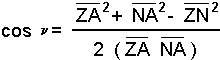Once angle v is found, the only question is whether to add it or subtract it from. In the example star A, simple addition will work. But this will not work for either of the other stars. The formulae we seek needs to be more refined.

The examples in graph 4.0.2 show that stars A and B are in the hemisphere defined as the Earth pole N lies to the right of the galactic pole Z. Any stars in this so defined hemisphere require an addition of the angleand angle v to find G.

`+ v = G4.1.3`
(With the example star B, the simple addition will work, if one keeps in mind that any amount of degrees over 360, a full circle, results in a number x° which can also be accurately defined as x° - 360°.)
Star C is in the hemisphere where N is to the left of Z and a subtraction is required;
`- v = G4.1.4`
If one examines the situation of star C closely, it can be seen thatis about 20°, while Gwould be about 310°. But trying to get this answer from formula 4.1.4 is not possible. The angle v for star C (angle NCZ) can be found using formula 4.1.2. Let us say it is 70°. Substituting values for formula 4.1.4 gives us:

`                         20° - 70° = -50°                  4.1.5`
Clearly we need even more refined formulae that takes all the possibilities into consideration. Where it is understood, as with example star B, if one goes totally around the circle, i.e. greater than 360° the true value of the angle is never greater than 360°. By the same token, if the degree value is less than zero, as in the case of star C, the true angle may be restated as a positive value:

`                      360 ° + -50° = 310°                  4.1.6`
The set of formulae to find the angle of Proper Motion based on galactic polar, Gcan be stated as:

`                                                            4.1.7`
Solutions to G(Hemisphere)
Position of Star based on given RA
For this value Do this formula
(N to the right of Z)
(- 180°) < RA+ v < 360° G+ v+ v = 360° G= v+ v > 360° G= 360° - (+ v)
(N to the left of Z)> RA > (-180°)- v < 0° G= 360° - (- v)- v = 0° G= v- v > 0° G+ v
(The star is on the circle described by N and Z)= RA, or RA = (-180°)
G= 0°

Where RA = Earth polar Right Ascension of the star,= the Earth polar angle of Right Ascension of the galactic north pole (a known value), v = the angle subended by the star and N and Z= Stellar angle of Proper Motion based on Earth polar.

#### Forward to Chapter 5 - Annual Shifts

Backward to Chapter 3 - The Time Coordinate